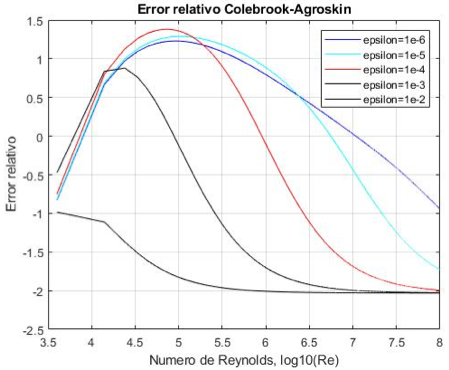# ON THE EXPLICIT EXPRESSIONS FOR THE DETERMINATION OF THE FRICTION FACTOR IN TURBULENT REGIME

• J.R. Pérez-Pupo
• M.N. Navarro-Ojeda
• J.N. Pérez-Guerrero
• M.A. Batista-Zaldívar
Keywords: hydraulic friction coefficient, turbulent regime, explicit models, relative roughness, explicit correlations

### Abstract

Pressure drop in pipelines is commonly calculated by the Darcy-Weisbach formula. To use this formula, the Darcy´s hydraulic friction factor must be known. The best approximation for the Darcy´s friction coefficient in turbulent flow regime, is expressed by the Colebrook-White equation. This equation can only be solved by numerical methods, because it is implicit for the friction coefficient. There are other approximate explicit models for the calculation with different relative errors in comparison to the Colebrook-White equation. In this work, a review of the friction factor explicit correlations is made including, for the first time in an article of this type, 48 equations, 10 of them that had not been reported previously and other explicit equations whose precision does not agree with previous reports or had not been included in review articles on this topic. The precision of these equations was determined by means of the maximum relative error for Reynold´s numbers and relative roughness, considered as the equation´s application range.Published
2019-06-15
How to Cite
Pérez-Pupo, J., Navarro-Ojeda, M., Pérez-Guerrero, J., & Batista-Zaldívar, M. (2019). ON THE EXPLICIT EXPRESSIONS FOR THE DETERMINATION OF THE FRICTION FACTOR IN TURBULENT REGIME. Revista Mexicana De Ingeniería Química, 19(1), 313-334. https://doi.org/10.24275/rmiq/Fen497
Section
Transport phenomena